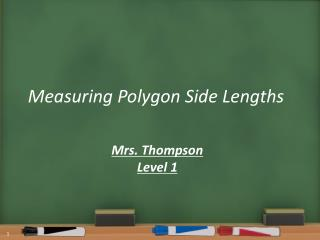DownloadDownload PresentationMeasuring Polygon Side Lengths

# Measuring Polygon Side Lengths

Download Presentation## Measuring Polygon Side Lengths

- - - - - - - - - - - - - - - - - - - - - - - - - - - E N D - - - - - - - - - - - - - - - - - - - - - - - - - - -
##### Presentation Transcript

1. Measuring Polygon Side Lengths Mrs. Thompson Level 1

2. Lesson Overview (4 of 4)

3. Warm Up CONTENT OBJECTIVE: You will be able to measure the side lengths of a polygon on the coordinate plane without counting. LANGUAGE OBJECTIVE: Students will talk about the x- and y-coordinates of points. Find the length of the line segment. Then write a paragraph explaining how you found the line segment. Be specific and include all details. Version 2 Answer

4. Agenda: CONTENT OBJECTIVE: You will be able to measure the side lengths of a polygon on the coordinate plane without counting. LANGUAGE OBJECTIVE: Students will talk about the x- and y-coordinates of points. 1) Warm Up (5 min, individual): Explain how to measure side length 2) Launch (15 min, whole class): New method for measuring side length 3) Explore/Practice (25 min, partner): Using both methods 4) Summary (5 min, whole class): Why (and when) use the new method? 5) Assessment (5 min, individual): Exit ticket

5. Handout: Launch, p.1 2 units Agenda

6. Handout: Launch, p.1 3 units Agenda

7. Handout: Launch, p.1 4 units Agenda

8. Handout: Launch, p.1 Questions: 1. What coordinate stays the same in each of the three examples? 2. What coordinate changes in each of the three examples? 3. What is the relationship between the coordinate that changes and the length of the line segment? (1,2) (3,2) (1,2) (3,2) 2 units 3 – 1 = 2 units Scaffold Agenda

9. Handout: Launch, p.1 Questions: 1. What coordinate stays the same in each of the three examples? 2. What coordinate changes in each of the three examples? 3. What is the relationship between the coordinate that changes and the length of the line segment? Answer Agenda

10. Handout: Launch, p.1 3 – 1 = 2 2 units Agenda

11. Handout: Launch, p.1 4 – 1 = 3 3 units Agenda

12. Handout: Launch, p.1 5 – 1 = 4 4 units Agenda

13. Handout: Launch, p.2 4 units Agenda

14. Handout: Launch, p.2 3 units Agenda

15. Handout: Launch, p.2 2 units Agenda

16. Handout: Launch, p.2 Questions: 1. What coordinate stays the same in each of the three examples? 2. What coordinate changes in each of the three examples? 3. What is the relationship between the coordinate that changes and the length of the line segment? (2,4) (2,4) 4 units (2,0) (2,0) 4 – 0 = 4 units Scaffold Agenda

17. Handout: Launch, p.2 Questions: 1. What coordinate stays the same in each of the three examples? 2. What coordinate changes in each of the three examples? 3. What is the relationship between the coordinate that changes and the length of the line segment? Answer Agenda

18. Handout: Launch, p.2 4 – 0 = 4 4 units Agenda

19. Handout: Launch, p.2 3 – 0 = 3 3 units Agenda

20. Handout: Launch, p.2 2 – 0 = 2 2 units Agenda

21. Handout: Launch, p.3 Summary: Another way to measure a horizontal or vertical line segment is by… Agenda Answer

22. Handout: Launch, p.3 Agenda Answer

23. Handout: Practice Page 1 Page 2 Page 3 Agenda

24. Handout: Practice, p.1 Polygon D Agenda Answer

25. Handout: Practice, p.2 2. Give the coordinates of three points that lie on a horizontal line with the point (–2, 7). Explain how you know that all three points lie on the same line with (–2,7). 3. Give the coordinates of three points that lie on a vertical line with the point (–2, 7). Explain how you know that all three points lie on the same line with (–2,7). Answer Agenda

26. Handout: Practice, p.4 Set #3 Perimeter is ________________. Answer Agenda

27. Handout: Practice, p.4 6. You are told that a rectangle has vertices at the following coordinates: To find the side lengths of the rectangle would you rather use Method #1: Counting on the Coordinate Plane or Method #2: Calculating Using Coordinates? Explain why you would use that method. (Note: you do not need to find the side lengths, just explain which strategy you would prefer and why) Agenda

28. Handout: Exit Ticket 1) Individually work on the exit ticket. 2) When you turn in your exit ticket, collect a homework handout. 3) Have a great day! Agenda